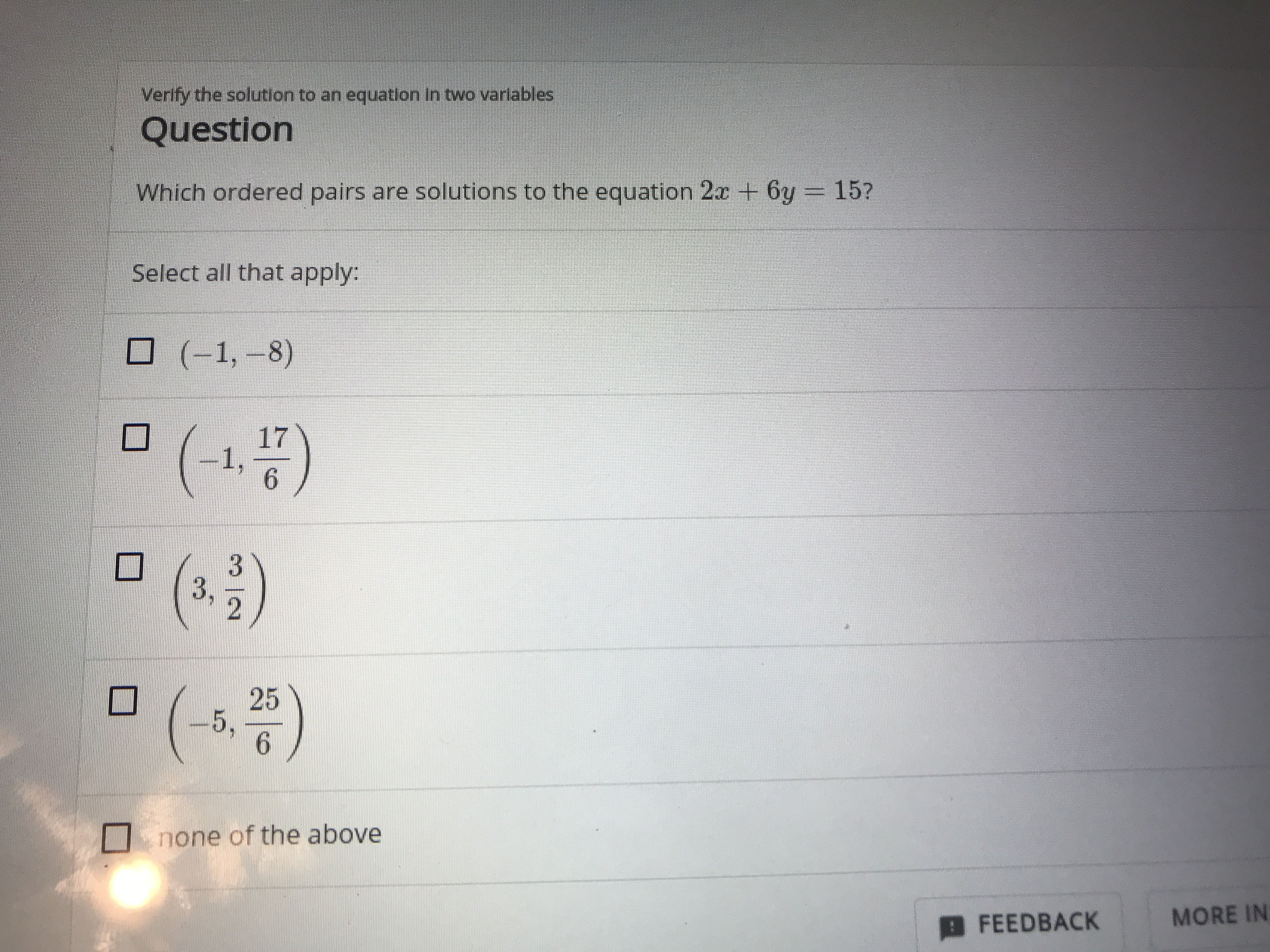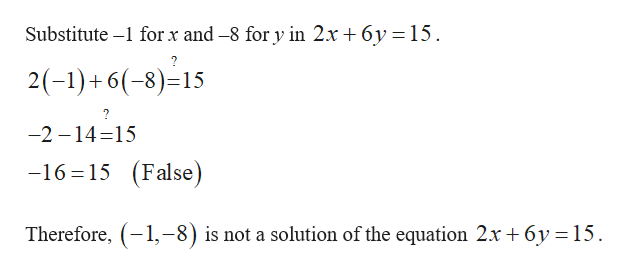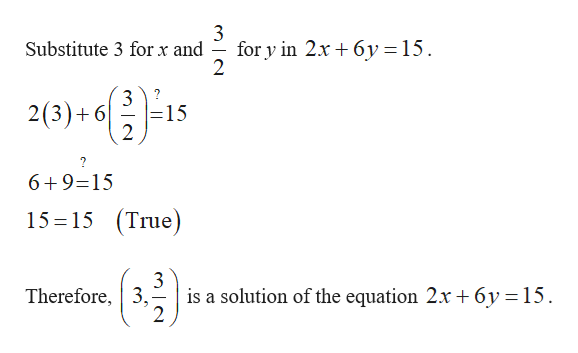# Verify the solution to an equation in two varlablesQuestionWhich ordered pairs are solutions to the equation 2ac 6y= 15?Select all that apply:(-1,-8)()171,63()255,6none of the aboveFEEDBACKMORE IN

Question
204 viewshelp_outlineImage TranscriptioncloseVerify the solution to an equation in two varlables Question Which ordered pairs are solutions to the equation 2ac 6y = 15? Select all that apply: (-1,-8) () 17 1, 6 3 () 25 5, 6 none of the above FEEDBACK MORE IN fullscreen
check_circle

Step 1

Verify whether (–1, –8) is solution to the equation 2x+6y=15.help_outlineImage TranscriptioncloseSubstitute - for x and -8 for y in 2x + 6y = 15. 2(-1)+6(-8)=15 ? -2 14-15 -16 15 (False) Therefore, 1,-8) is not a solution of the equation 2x 6y 15 fullscreen
Step 2

Verify whether (–1 ,17/6) is solution to the equation 2x+6y=15.

Step 3

Verify whether (3 ,3/2) is solution to the ...help_outlineImage Transcriptionclose3 for y in 2x 6y = 15 2 Substitute 3 for x and 3? 2(3)6 - 15 2 6+9-15 (True) 15 15 Therefore, 3, is a solution of the equation 2x +6y = 15 2 fullscreen

### Want to see the full answer?

See Solution

#### Want to see this answer and more?

Solutions are written by subject experts who are available 24/7. Questions are typically answered within 1 hour.*

See Solution
*Response times may vary by subject and question.
Tagged in